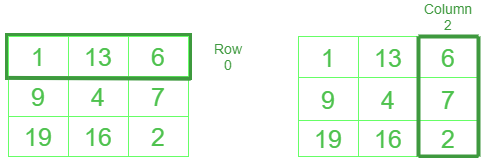How to access a NumPy array by column

• Difficulty Level : Easy
• Last Updated : 29 May, 2021

Accessing a NumPy based array by a specific Column index can be achieved by the indexing. Let’s discuss this in detail.

NumPy follows standard 0 based indexing.Row and column in NumPy are similar to Python List

Examples:

Given array : 1 13 6
9  4 7
19 16 2

Input: print(NumPy_array_name[ :,2])

# printing 2nd column
Output: [6 7 2]

Input: x =  NumPy_array_name[ :,1]
print(x)

# storing 1st column into variable x
Output:  [13 4 16]

Method #1: Selection using slices

Syntax :

For column : numpy_Array_name[  : ,column]

For row : numpy_Array_name[ row, :  ]

Python3

 # Python code to select row and column# in NumPy import numpy as np array = [[1, 13, 6], [9, 4, 7], [19, 16, 2]] # defining arrayarr = np.array(array) print('printing array as it is')print(arr) print('printing 0th row')print(arr[0, :]) print('printing 2nd column')print(arr[:, 2]) # multiple columns or rows can be selected as wellprint('selecting 0th and 1st row simultaneously')print(arr[:,[0,1]])

Output :

printing array as it is
[[ 1 13  6]
[ 9  4  7]
[19 16  2]]
printing 0th row
[ 1 13  6]
printing 2nd column
[6 7 2]
selecting 0th and 1st row simultaneously
[[ 1 13]
[ 9  4]
[19 16]]

Method #2: Using Ellipsis

Syntax :

For column : numpy_Array_name[,column]

For row : numpy_Array_name[row,]

where ‘‘ represents no of elements in the given row or column

Note: This is not a very practical method but one must know as much as they can.

Python3

 # program to select row and column# in numpy using ellipsis import numpy as np # defining arrayarray = [[1, 13, 6], [9, 4, 7], [19, 16, 2]] # converting to numpy arrayarr = np.array(array) print('printing array as it is')print(arr) print('selecting 0th column')print(arr[..., 0]) print('selecting 1st row')print(arr[1, ...])

Output :

printing array as it is
[[ 1 13  6]
[ 9  4  7]
[19 16  2]]
selecting 0th column
[ 1  9 19]
selecting 1st row
[9 4 7]

My Personal Notes arrow_drop_up